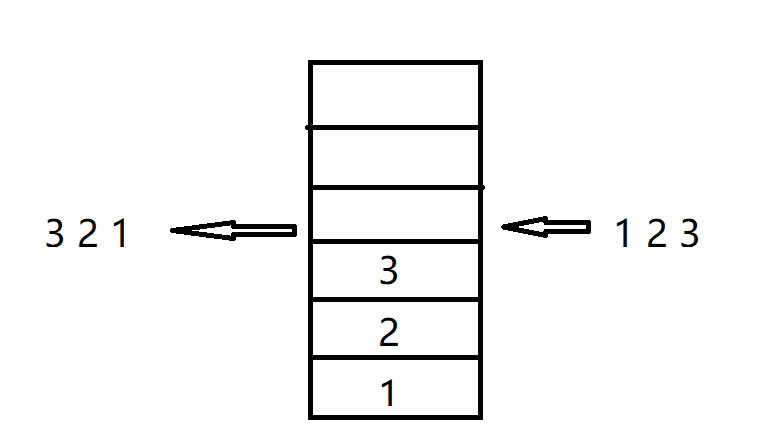【注意】 本文档存在大量的不规范不标准言论以及大量臆想，请勿作为学术参考

# 有关栈数据处理和布尔代数的探索

## 先进后出的栈OK，那么我们就得到了定理一（请原谅我这么称呼）

## 逻辑的混乱

$$\LARGE C_{2n}^{n}-C_{2n}^{n-1}$$

$$\LARGE C_{24}^{4}-C_{24}^{4-1}=14$$

• 单数据块进栈
• 双数据块进栈
• 3数据块进栈
• 4数据块进栈

3数据块 ab c d \ a bc d \ a b cd a bc d \ bacd \ abdc
4数据块 a b c d dcba

## 于迷雾中出现的布尔代数

$$\LARGE \overline{ab} = ba$$

• # 4数据块$$\LARGE\overline{a},\overline{b},\overline{c},\overline{d}, abcd$$
如此一来，进栈出栈的数据运算被我们抽象为了逻辑运算，栈结构的数据操作，奇迹般的和布尔代数联系到了一起！实际上这一步抽象是对栈结构的二元性的抽象应用。这让我不禁回想起了某个开发者朋友和我说过的一句话

$$\LARGE\overline{\overline{ab}c}\overline{d}$$

## 不同寻常的逻辑门

$$\LARGE\overline{a},\overline{b} = ab$$

$$\LARGE\overline{a}\wedge\overline{b} = ab$$

$$\LARGE\overline{a}\vee\overline{b} = ba$$

$\wedge$ 连接的两个数据块顺序输出，$\vee$ 连接的两个数据块逆序输出

$$\LARGE\overline{\overline{ab}c}\overline{d}=\overline{\overline{a\wedge b}\wedge c}\wedge \overline{d}$$

## 神奇的德摩根定律

$$\LARGE\overline{a\wedge b}=\LARGE\overline{a}\vee\overline{b}$$

$$\LARGE\overline{\overline{ab}c}\overline{d}=\overline{\overline{a\wedge b}\wedge c}\wedge \overline{d}=\overline{(a\vee b)\wedge c}\wedge\overline{d}=(a\wedge b)\vee c\wedge d=cabd$$

$$\LARGE\overline{\overline{ab},\overline{cd}}=cdab$$

3数据块 $\large\overline{\overline{ab},\overline{cd}}\quad\overline{a\overline{bc}d}$ $\large cdab\quad dbca$